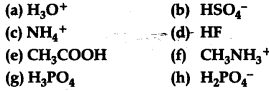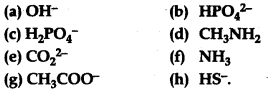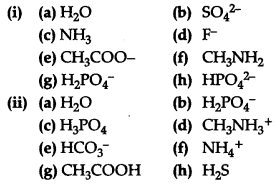# (i)State the formula and name of the conjugate base of each of the following acids:

(i)State the formula and name of the conjugate base of each of the following acids:(ii)State the formula and name of the conjugate acid of each of the following bases: